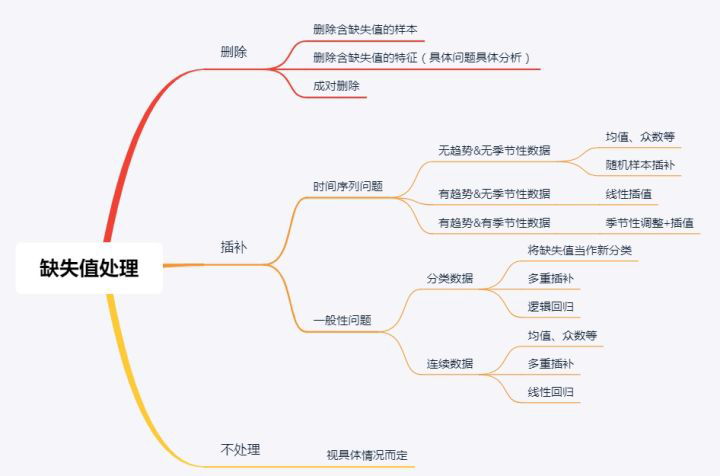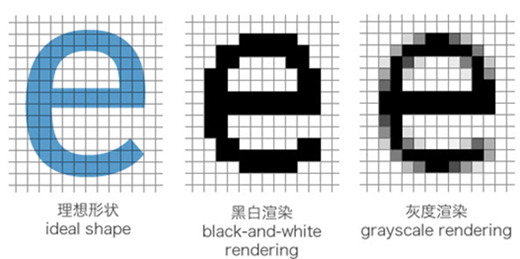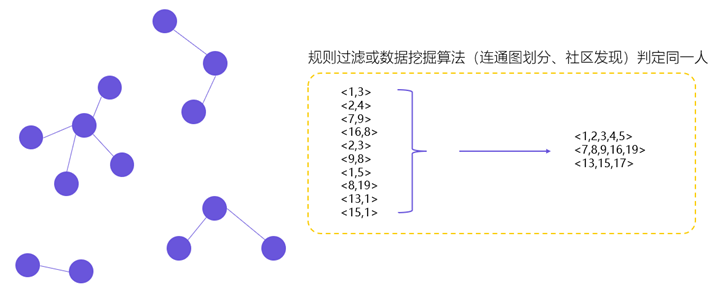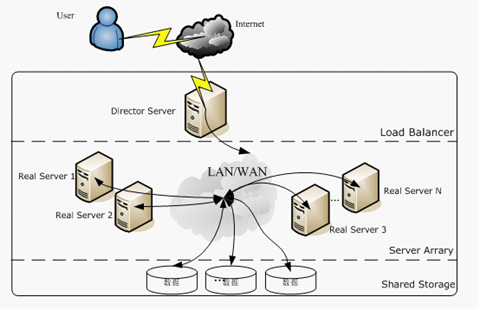机器学习/数据分析之缺失值处理

数据缺失类型

• 完全随机丢失（MCAR，Missing Completely at Random）：某个变量是否缺失与它自身的值无关，也与其他任何变量的值无关。例如，由于测量设备出故障导致某些值缺失。
• 随机丢失（MAR，Missing at Random）： 在控制了其他变量已观测到的值后，某个变量是否缺失与它自身的值无关。例如，人们是否透露收入可能与性别、教育程度、职业等因素有关系。
• 非随机丢失（MNAR，Missing not at Random）：数据的缺失与不完全变量自身的取值有关。分为两种情况：缺失值取决于其假设值（例如，高收入人群通常不希望在调查中透露他们的收入）；或者，缺失值取决于其他变量值（假设女性通常不想透露她们的年龄，则这里年龄变量缺失值受性别变量的影响）。

缺失值处理缺失值统计

missing_value_formats = ["n.a.","?","NA","n/a", "na", "--"]
df = pd.read_csv("employees.csv", na_values = missing_value_formats)
df.isna().sum()

缺失值删除

• 可用于任何类型的统计分析。
• 不需要特别的运算方法。
• 如果数据是MCAR，则减少的样本将会是原样本的一个随机次样本。
• 如果任何因变量缺失数据的概率不取决于自变量的值，则使用成列删除的回归估计值将会是无偏误的。

• 标准误通常较大。
• 如果数据不是MCAR而只是MAR，那么成列删除可能会产生有偏误的估计值。(例如，教育缺失数据的概率取决于职业地位，那么对于二者的回归会产生一个有偏误的回归系数估计值。)

• 如果数据是MAR但不是随机被观察到的，估计值可能会严重偏误。
• 由统计软件所产生的标准误和检验统计量估计时偏误的。
• 在小样本中，建构的协方差或相关矩阵可能不是“正定的”。

Pandas中的dropna()方法

DataFrame.dropna(axis=0, how='any', thresh=None, subset=None, inplace=False)

• axis：轴。0或’index’，表示按行删除；1或’columns’，表示按列删除。
• how：筛选方式。‘any’，表示该行/列只要有一个以上的空值，就删除该行/列；‘all’，表示该行/列全部都为空值，就删除该行/列。
• thresh：非空元素最低数量。int型，默认为None。如果该行/列中，非空元素数量小于这个值，就删除该行/列。
• subset：子集。列表，元素为行或者列的索引。如果axis=0或者‘index’，subset中元素为列的索引；如果axis=1或者‘column’，subset中元素为行的索引。由subset限制的子区域，是判断是否删除该行/列的条件判断区域。
• inplace：是否原地替换。布尔值，默认为False。如果为True，则在原DataFrame上进行操作，返回值为None。

# 删除所有含空的行
df.dropna(inplace=True)

# 删除某列含控制的行
df.dropna(subset=['列名'],inplace=True)

缺失值插补

• 统计法：对于数值型的数据，使用均值、加权均值、中位数等方法补足；对于分类型数据，使用类别众数最多的值补足。
• 模型法：更多时候我们会基于已有的其他字段，将缺失字段作为目标变量进行预测，从而得到最为可能的补全值。如果带有缺失值的列是数值变量，采用回归模型补全；如果是分类变量，则采用分类模型补全。
• 专家补全：对于少量且具有重要意义的数据记录，专家补足也是非常重要的一种途径。
• 其他方法：例如随机法、特殊值法、多重填补等。

K最近距离邻法（K-means clustering）

• 连续数据：最常用的距离度量有欧氏距离，曼哈顿距离以及余弦距离。
• 分类数据：汉明（Hamming）距离在这种情况比较常用。对于所有分类属性的取值，如果两个数据点的值不同，则距离加一。汉明距离实际上与属性间不同取值的数量一致。

KNN算法最吸引人的特点之一在于，它易于理解也易于实现。其非参数的特性在某些数据非常“不寻常”的情况下非常有优势。

KNN算法的一个明显缺点是，在分析大型数据集时会变得非常耗时，因为它会在整个数据集中搜索相似数据点。此外，在高维数据集中，最近与最远邻居之间的差别非常小，因此KNN的准确性会降低。

EM算法是一种在不完全数据情况下计算极大似然估计或者后验分布的迭代算法。在每一迭代循环过程中交替执行两个步骤：

• E步（Excepctaion step，期望步），在给定完全数据和前一次迭代所得到的参数估计的情况下计算完全数据对应的对数似然函数的条件期望
• M步（Maximzation step，极大化步），用极大化对数似然函数以确定参数的值，并用于下步的迭代。

1. 为每个空值产生一套可能的插补值，这些值反映了无响应模型的不确定性；每个值都可以被用来插补数据集中的缺失值，产生若干个完整数据集合。
2. 每个插补数据集合都用针对完整数据集的统计方法进行统计分析。
3. 对来自各个插补数据集的结果，根据评分函数进行选择，产生最终的插补值。

• 贝叶斯估计以极大似然的方法估计，极大似然的方法要求模型的形式必须准确，如果参数形式不正确，将得到错误得结论，即先验分布将影响后验分布的准确性。而多重插补所依据的是大样本渐近完整的数据的理论，在数据挖掘中的数据量都很大，先验分布将极小的影响结果，所以先验分布的对结果的影响不大。
• 贝叶斯估计仅要求知道未知参数的先验分布，没有利用与参数的关系。而多重插补对参数的联合分布作出了估计，利用了参数间的相互关系。

• 为表现数据分布，随机抽取进行插补，增加了估计的有效性。
• 当多重插补是在某个模型下的随机抽样时，按一种直接方式简单融合完全数据推断得出有效推断，即它反映了在该模型下由缺失值导致的附加变异。
• 在多个模型下通过随机抽取进行插补，简单地应用完全数据方法，可以对无回答的不同模型下推断的敏感性进行直接研究。

• 生成多重插补比单一插补需要更多工作
• 贮存多重插补数据集需要更多存储空间
• 分析多重插补数据集比单一插补需要花费更多精力

C4.5方法

Pandas中的fillna方法

DataFrame.fillna(value=None,method=None,axis=None,inplace=False,limit=None,downcast=None,**kwargs)

• value：接收常数、dict、Series 或 DataFrame，表示填充缺失值的值。
• axis：指定填充方向，当 axis=1 按列填充，axis=0 按行填充。
• inplace：接收 True 或 False。True 表示直接修改原对象，False 表示创建一个副本，修改副本，原对象不变，默认为 False。
• limit：表示限制填充的个数，如果 limit=2，则只填充两个缺失值。
• downcast：默认为 None，如果需要将填充的值向下转换为适当的相等数据类型的数值，如将 float64 数据类型转换为 int64 数据类型时，则此参数的值为 ‘infer’。

# 用常量0填充
df1 = df.fillna(0)

# 用字典填充
df2 = df.fillna({'Normal':60,'exam':40})

df2 = df.fillna(method='ffill')

# 将exam列的缺失值用均值替换
exa_mea = df['exam'].fillna(df['exam'].mean())

# 将Normal列的缺失值用中位数替换
Nor_med = df['Normal'].fillna(df['Normal'].median())

# 使众数（mode）填充
df['Normal'].fillna(df['Normal'].mode())

# 使用随机方式填充
df["column"].fillna(lambda x: random.choice(df[df[column] != np.nan]["column"]), inplace =True)

Pandas中的interpolate()方法

Series 和 DataFrame 对象都有interpolate()方法，默认情况下，该函数在缺失值处执行线性插值。这个方法是利用数学方法来估计缺失点的值，对于较大的数据非常有用。

DataFrame.interpolate(self, method='linear', axis=0, limit=None, inplace=False, limit_direction='forward', limit_area=None, downcast=None, **kwargs)

• method ：str，默认为linear，差值方法可选参数:
• linear：忽略索引，并将值等距地对待。这是MultiIndexes支持的唯一方法。
• time：处理每日和更高分辨率的数据，以内插给定的时间间隔长度。
• index，values：索引，值，使用索引的实际数值
• nearest, zero, slinear, quadratic, cubic, spline, barycentric, polynomial：传递给interpolate.interp1d，这些方法使用索引的数值。polynomial 和 spline都要求您还指定一个顺序(int)，例如 df.interpolate(method=’polynomial’，order=5)
• nearest：最近
• zero：零
• slinear：线性
• cubic：立方
• spline：花键，样条插值
• barycentric：重心插值
• polynomial：多项式
• krogh, piecewise_polynomial, spline, pchip, akima: SciPy 类似名称的插值方法。
• krogh: 克罗格插值
• piecewise_polynomial: 分段多项式
• spline: 样条插值
• pchip: 立方插值
• akima: 阿克玛插值
• from_derivatives：指interpolate.BPoly.from_derivatives，它替换了 scipy 0.18 中的 piecewise_polynomial 插值方法。
• axis: 插值应用的轴方向，可选择 {0 or index, 1 or columns, None}, 默认为 None
• limitint: 要填充的连续 NaN 的最大数量， 必须大于 0。
• inplace: 是否将最终结果替换原数据，默认为 False
• limit_direction: 限制方向，可传入 {forward, backward, both}, 默认forward，如果指定了限制，则将沿该方向填充连续的 NaN
• limit_area: 限制区域，可传入 {None, inside, outside}, 默认 None，如果指定了限制，则连续的NaN将被此限制填充
• None: 没有填充限制
• inside: 仅填充有效值包围的NaN（内插）
• outside: 仅将NaN填充到有效值之外（外推）
• downcast: 可传入‘infer’ 或者 None, 默认是 None，如果可以向下转换 dtypes
• **kwargs: 传递给插值函数的关键字参数

• 邻近点插值（method=’nearest’）。
• 线性插值（method=’linear’）：在两个数据点之间连接直线，计算给定的插值点在直线上的值作为插值结果，该方法是interp1函数的默认方法。
• 三次样条插值（method=’spline’）：通过数据点拟合出三次样条曲线，计算给定的插值点在曲线上的值作为插值结果。
• 立方插值（method=’pchip’ or ‘cubic’）：通过分段立方Hermite插值方法计算插值结果。

• 邻近点插值方法的速度最快，但平滑性最差；
• 线性插值方法占用的内存较邻近点插值方法多，运算时间也稍长，与邻近点插值不同，其结果是连续的，但顶点处的斜率会改变；
• 三次样条插值方法的运算时间最长，但内存的占用较立方插值法要少，但其插值数据和导数都是连续的。在这4种方法中，三次样条插值结果的平滑性最好，但如果输入数据不一致或数据点过近，就可能出现很差的插值效果。

def fill_dependents_missing(data, to_fill):
df = data.copy()
columns = [*df.columns]
columns.remove(to_fill)

X = df.loc[:, columns]
y = df.loc[:, to_fill]
X_train = X.loc[df[to_fill].notnull()]
y_train = y.loc[df[to_fill].notnull()]
X_pred = X.loc[df[to_fill].isnull()]
rfr = RandomForestRegressor(random_state=22, n_estimators=200, max_depth=3, n_jobs=-1)
rfr.fit(X_train, y_train)
y_pred = rfr.predict(X_pred).round()
df.loc[df[to_fill].isnull(), to_fill] = y_pred
return df

不处理缺失值

• 忽略，缺失值不参与距离计算，例如KNN。
• 将缺失值作为分布的一种状态，并参与到建模过程，例如各种决策树及其变体。
• 不基于距离做计算，因此基于值的距离做计算本身的影响就消除了，例如DBSCAN。网站与APP开发中的字体设置用户体系搭建之ID-Mapping